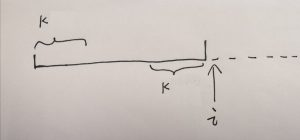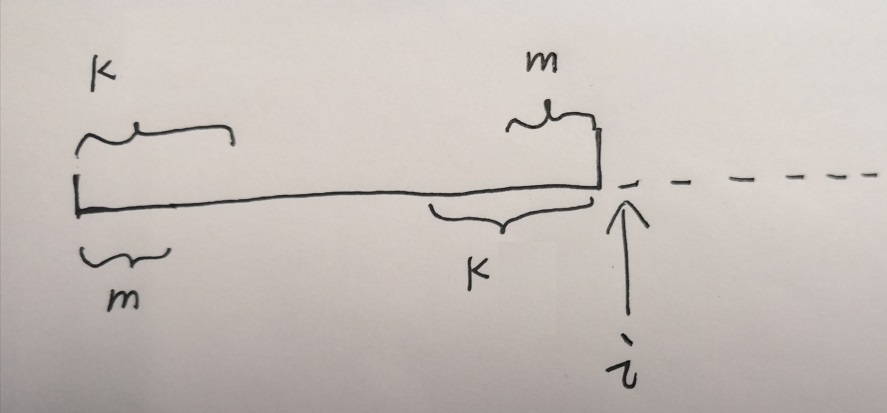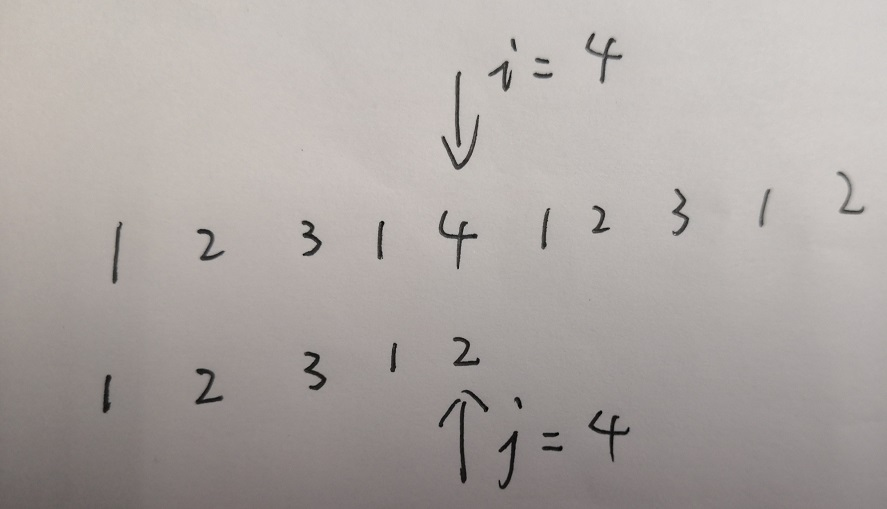• KMP 中的前缀后缀

• 构建 Next数组

• KMP算法匹配过程

• 源码示例.

## KMP 中的前缀后缀

KMP 中需要预先计算字符串 最长相同前后缀的长度，用于加快比对过程，具体原理第三部分详细说明。

## 如何构建 Next 数组## KMP 算法过程## 完整实现

string match = "kmpmmkmpm";

vector<int> makeNext(string &base){
vector<int> next(base.size(), 0);
for(int i = 1; i < base.size(); ++i){
int k = next[i - 1];
while(k > 0 && base[k] != base[i])
k = next[k - 1];

if (base[k] == base[i])
next[i] = ++k;
}
return next;
}

vector<int> kmpMatch(string &input, string &match){
vector<int> next = makeNext(match);
vector<int> matchIndexs;
// i 为 input下标, j 为 match下标
int i = 0, j = 0;
while (i < input.size()){
if (input[i] == match[j]){
++i;
++j;
if (j == match.size()){
matchIndexs.push_back(i - match.size());
j = next[j - 1];
}
}else{
if (j == 0)
++i;
else
j = next[j - 1];
}
}
return matchIndexs;
}